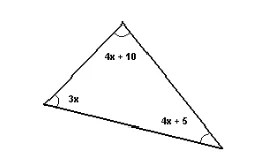Wednesday , September 29 2021
Breaking News

# NTS Quantitative Reasoning Practice Test 3

### NTS Quantitative Reasoning Practice Test # 3

INSTRUCTIONS:

• This Quiz is related to NTS Quantitative Reasoning Practice Test # 3 in this series
• The Quiz based on the Topic, “Quantitative Reasoning
• If you have good understanding about the subject, then you can attempt it
• There will be 10 Multiple Choice Questions (MCQs) in this test
• You have 60 Seconds to answer a single Multiple Choice Question
• Questions will be randomly changed every time you start this test
• You should practice more and more to get high marks
• Passing Criteria is 60 percent for this Quiz
• You can retake this test as many time as you like
• The progress bar at the top of screen will show your progress as well as the time remaining where timed quiz
• After the quiz, You will find Your Test Score and Grade
• If you feel that any Incorrect Answer to a Question, simply Comment us about the Question.

#### The total area of the shape below is equal to 240. Find the length of segment AC.Correct! Wrong!

Let us express the total area as the area of the upper and lower triangles with commom base AC (1/2)(20)(AC) + (1/2)(28)(AC) = 240 Multiply all terms by 2, simplify and solve for AC (20)(AC) + (28)(AC) = 480 48 AC = 480 AC = 480 / 48 =10

#### Find the values of the constants k and m so that the equation 2 x2 + kx - m = 0 has solutions at x = 1 and x = -2. ​

Correct! Wrong!

Since x = 1 and x = -2 are solutions to the equation, they must satisfy the equation 2(1)2 + k(1) - m = 0 and 2(-2)2 + k(-2) - m = 0 Simplify and rewrite as a system of two equations in two unknowns k and m. k - m = - 2 and -2k - m = -8 Subtract the left and right hand terms of the two equations to obtain an equivalent equation. (k - m) - (-2k - m) = (-2) - (-8) Simplify. 3k = 6 Solve for k. k = 2 and Use equation k - m = - 2 to find m. m = k + 2 = 4 The values of k and m are k = 2 and m = 4

#### Find x ?Correct! Wrong!

The sum of all 3 angles of any triangle is equal to 180 degrees. Hence (4x + 10) + (4x + 5) + (3x) = 180 Group like terms and solve for x 11x = 165 or x = 15

#### If 3x + 5y = 5 and x + 3y = 20, then 2x + 4y =

Correct! Wrong!

Add the left and right hand sides of the given equations to obtain a new equation (3x + 5y) + (x + 3y) = (5) + (20) Simplify 4x + 8y = 25 Divide all taerms of the above equation by 2 2x + 4y = 25 / 2

#### Which of the following has the greatest value?

Correct! Wrong!

Use decimal numbers to rewrite the above expressions A) 250% = 250/100 = 2.5 B) 2 + 1/2 = 2 + 0.5 = 2.5 C) 5 × 0.5 = 2.5 D) 1 / 0.1 = 10 The expression 1 / 0.1 has the greatest value.

#### Find the values of the constants b and c so the equation x2 + bx + c = 0 has solutions at x = 2 and x =

Correct! Wrong!

Since the equation has solutions at x = 2 and x = - 1, it can be written in factored form as follows (x - 2)(x + 1) = 0 Expand the left hand side x2 - x - 2 = 0 If we now compare the given equation x2 + bx + c = 0 with the obtained equation x2 - x - 2 = 0, we can identify constants b and c by their values as follows b = -1 and c = -2.

#### The measures, in degrees, of two consecutive interior angles M and N of a parallelogram are given by 2x + 10 and x + 20 respectively. Find the measures of the two angles.

Correct! Wrong!

Any two consecutive angle of a parallelogram are supplementary. Hence (2x + 10) + (x + 20) = 180 Solve for x 3x + 30 = 180 x = 50 We now evaluate M and N as follows M = 2x + 10 = 2(50) + 10 = 110° N = x + 20 = 50 + 20 = 70°

#### (4x2 - (- 4/3)(x + 1)4) / (- 3x + 3) =

Correct! Wrong!

Factor numerator as follows (4x2 - 4) = 4(x2 - 1) = 4(x - 1)(x + 1) Factor denominator as follows (- 3x + 3) = - 3(x - 1) Substitute in the given expression and simplify (4x2 - 4) / (- 3x + 3) = [ 4(x - 1)(x + 1) ] / [ - 3(x - 1)] = (- 4/3)(x + 1)

#### Solve the equation x2 + 5x = - 6 for x.

Correct! Wrong!

Solve for x x + 2 = 0 or x + 3 = 0 Solutions: x = - 2 and x = - 3

Correct! Wrong!